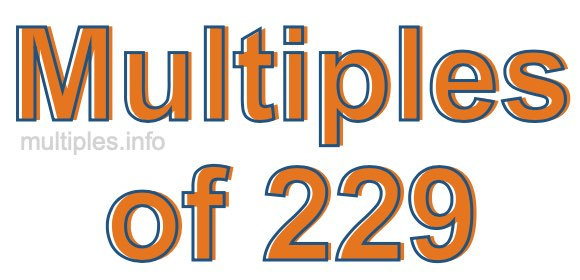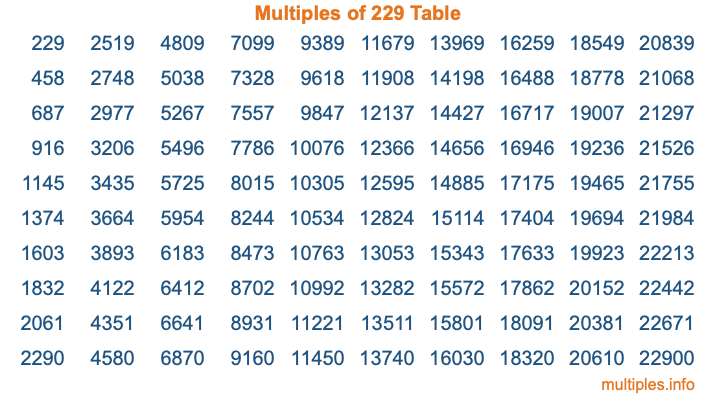Multiples of 229Welcome to the Multiples of 229 page. Here we will first teach you everything you will ever need to know about the multiples of 229, and then give you a study guide summary of everything we taught you to make sure you remember it all. Use this page to look up facts and learn information about the multiples of 229. This page will make you a multiples of two hundred twenty-nine expert!

Definition of Multiples of 229
Multiples of 229 are all the numbers that when divided by 229 equal an integer. Each of the multiples of 229 are called a multiple. A multiple of 229 is created by multiplying 229 by an integer.

Therefore, to create a list of multiples of 229, you start with 1 multiplied by 229, then 2 multiplied by 229, then 3 multiplied by 229, and so on for as long as you want. Thus, the list of the first five multiples of 229 is 229, 458, 687, 916, and 1145. To see a larger list of multiples of 229, see the printable image of Multiples of 229 further down on this page. We also have a category where you can choose any nth multiple of 229.

Multiples of 229 Checker
The Multiples of 229 Checker below checks to see if any number of your choice is a multiple of 229. In other words, it checks to see if there is any number (integer) that when multiplied by 229 will equal your number. To do that, we divide your number by 229. If the the quotient is an integer, then your number is a multiple of 229.

Is  a multiple of 229?

Least Common Multiple of 229 and ...
A Least Common Multiple (LCM) is the lowest multiple that two or more numbers have in common. This is also called the smallest common multiple or lowest common multiple and is useful to know when you are adding our subtracting fractions. Enter one or more numbers below (229 is already entered) to find the LCM.

Check out our LCM Calculator if you need more details about the Least Common Multiple or if you need the LCM for different numbers for adding and subtraction fractions.

nth Multiple of 229
As we stated above, 229 is the first multiple of 229, 458 is the second multiple of 229, 687 is the third multiple of 229, and so on. Enter a number below to find the nth multiple of 229.

th multiple of 229

Multiples of 229 vs Factors of 229
229 is a multiple of 229 and a factor of 229, but that is where the similarities end. All postive multiples of 229 are 229 or greater than 229. All positive factors of 229 are 229 or less than 229.

Below is the beginning list of multiples of 229 and the factors of 229 so you can compare:

Multiples of 229: 229, 458, 687, 916, 1145, etc.

Factors of 229: 1, 229

As you can see, the multiples of 229 are all the numbers that you can divide by 229 to get a whole number. The factors of 229, on the other hand, are all the whole numbers that you can multiply by another whole number to get 229.

It's also interesting to note that if a number (x) is a factor of 229, then 229 will also be a multiple of that number (x).

Multiples of 229 vs Divisors of 229
The divisors of 229 are all the integers that 229 can be divided by evenly. Below is a list of the divisors of 229.

Divisors of 229: 1, 229

The interesting thing to note here is that if you take any multiple of 229 and divide it by a divisor of 229, you will see that the quotient is an integer.

Multiples of 229 Table
Below is an image of the first 100 multiples of 229 in a table. The table is in chronological order, column by column. The first column has the first ten multiples of 229, the second column has the next ten multiples of 229, and so on.The Multiples of 229 Table is also referred to as the 229 Times Table or Times Table of 229. You are welcome to print out our table for your studies.

Negative Multiples of 229
Although not often discussed or needed in math, it is worth mentioning that you can make a list of negative multiples of 229 by multiplying 229 by -1, then by -2, then by -3, and so on, to get the following list of negative multiples of 229:

-229, -458, -687, -916, -1145, etc.

Multiples of 229 Summary
Below is a summary of important Multiples of 229 facts that we have discussed on this page. To retain the knowledge on this page, we recommend that you read through the summary and explain to yourself or a study partner why they hold true.

There are an infinite number of multiples of 229.

A multiple of 229 divided by 229 will equal a whole number.

229 divided by a factor of 229 equals a divisor of 229.

The nth multiple of 229 is n times 229.

The largest factor of 229 is equal to the first positive multiple of 229.

229 is a multiple of every factor of 229.

229 is a multiple of 229.

A multiple of 229 divided by a divisor of 229 equals an integer.

229 divided by a divisor of 229 equals a factor of 229.

Any integer times 229 will equal a multiple of 229.

Multiples of a Number
Here you can get the multiples of another number, all with the same attention to detail as we did for multiples of 229 on this page.

Multiples of
Multiples of 230
Did you find our page about multiples of two hundred twenty-nine educational? Do you want more knowledge? Check out the multiples of the next number on our list!